# AP Physics 1: Graphing Kinematics Chapter Exam

Exam Instructions:

Choose your answers to the questions and click 'Next' to see the next set of questions. You can skip questions if you would like and come back to them later with the yellow "Go To First Skipped Question" button. When you have completed the practice exam, a green submit button will appear. Click it to see your results. Good luck!

### Page 1

#### Question 1 1. On this graph, what is the change in velocity between 5 seconds and 8 seconds?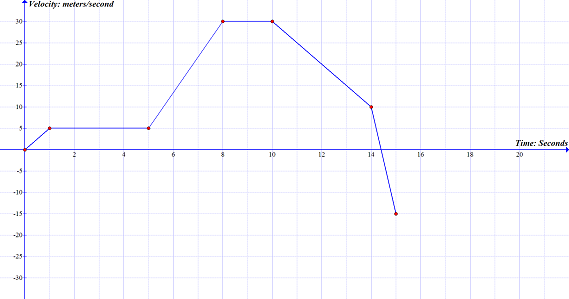#### Question 2 2. On this graph, what is the acceleration between 10 seconds and 14 seconds?### Page 2

#### Question 6 6. This graph represents how fast you jogged over a specific period of time. If you traveled 3 meters in 1 second, using the graph, what label should be at position A?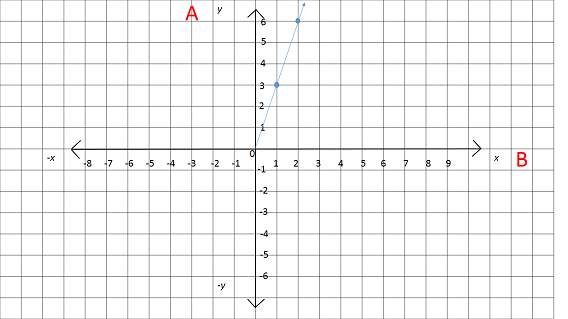#### Question 9 9. Using this graph, at what point is there a change in direction?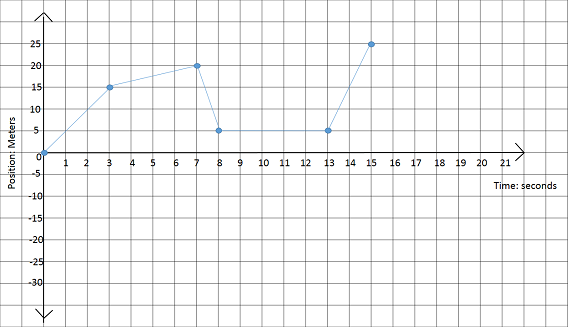#### Question 10 10. Using this graph, at what time is the object not moving?### Page 3

#### Question 13 13. For this graph, calculate the average speed between 12 seconds and 20 seconds.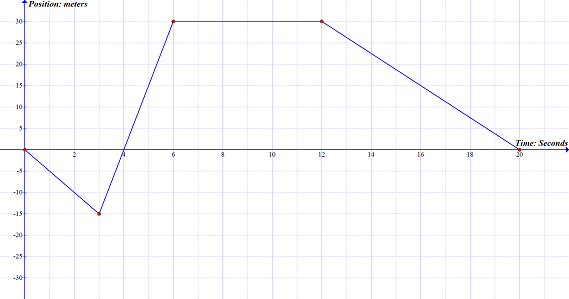#### Question 14 14. Calculate the slope of the line on the graph.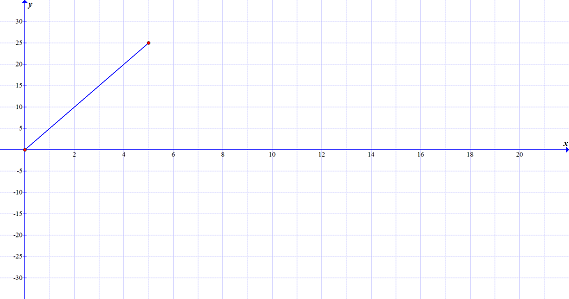#### Question 15 15. Using the graph, calculate the displacement for the section labeled A.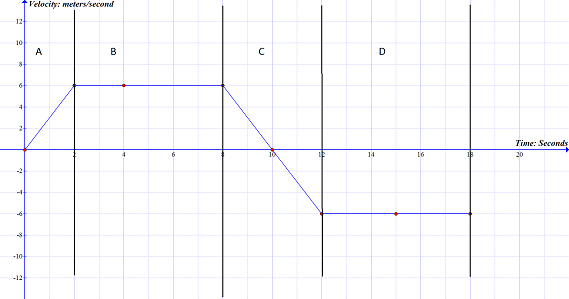### Page 4

#### Question 19 19. Which of the following correctly shows how to subtract vector b from vector a?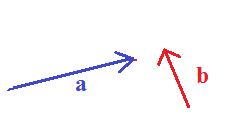### Page 5

#### Question 23 23. This graph is broken up into 5 sections, labeled A-E .On this graph, what section(s) represents negative acceleration?#### Question 24 24. This graph is broken up into 5 sections, labeled A-E. On this graph, what section(s) represents positive acceleration?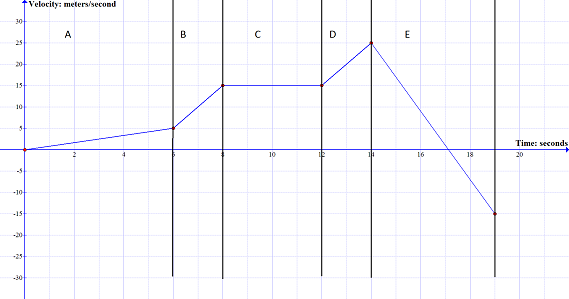### Page 6

#### Question 27 27. This graph represents an object that is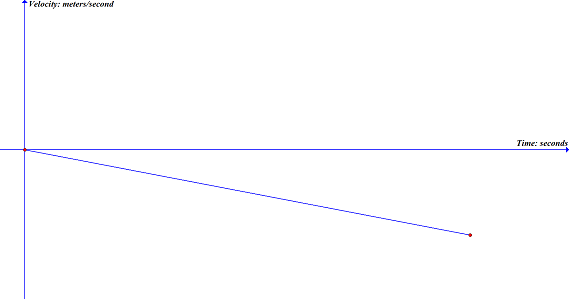#### Question 28 28. This graph represents an object that is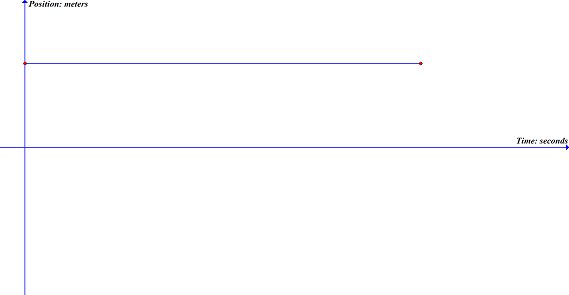#### AP Physics 1: Graphing Kinematics Chapter Exam Instructions

Choose your answers to the questions and click 'Next' to see the next set of questions. You can skip questions if you would like and come back to them later with the yellow "Go To First Skipped Question" button. When you have completed the practice exam, a green submit button will appear. Click it to see your results. Good luck!

Support